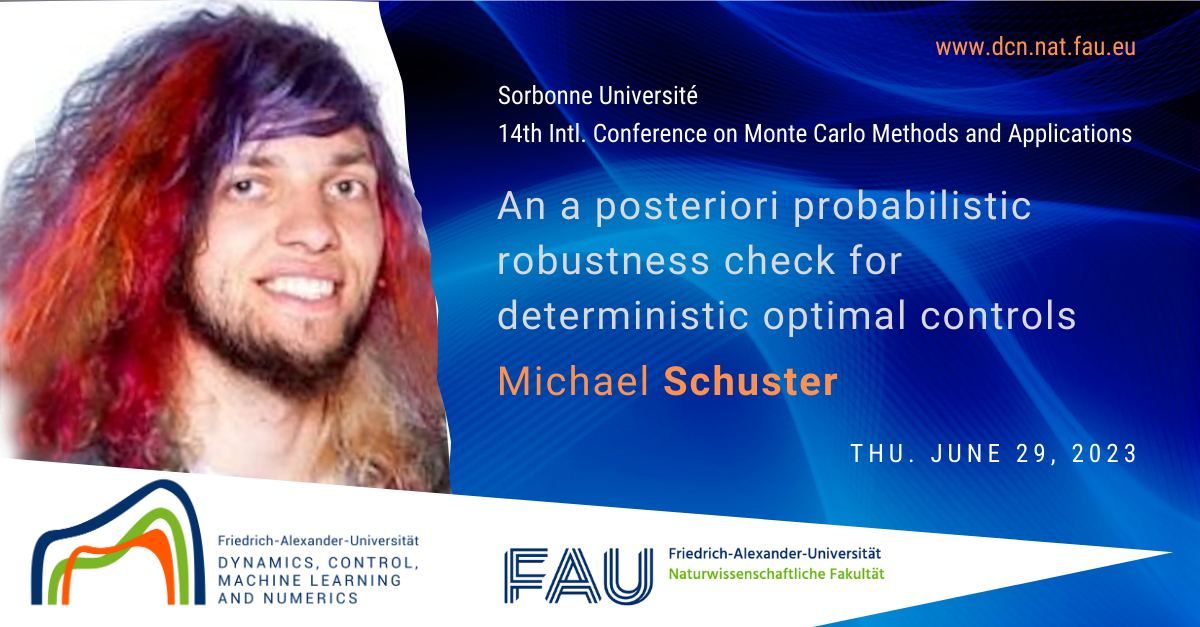# An a posteriori Probabilistic Robustness Check for Deterministic Optimal Controls

Next Thursday June 29, 2023 our postdoctoral researcher Michael Schuster, will present the poster on “An a posteriori Probabilistic Robustness Check for Deterministic Optimal Controls” at the 14th International Conference on Monte Carlo Methods and Applications organized on June 26 – 30, 2023 at the Sorbonne Université in Paris, France.

Abstract. We consider uncertain gas transport through pipeline networks and we propose a novel strategy to measure the robustness of determinstically computed compressor and valve controls. First, we consider a deterministic gas network problem and optimize the controls such that the total control cost is minimized subject to certain constraints on the pressure and gas flow. Then, we extend the gas network problem by uncertain gas demands, but maintain the determinstically computed control. We define the probabilistic robustness of the deterministic control as the probability that the pressure at certain locations in the gas network satisfies given bounds. Moreover, we introduce buffer zones in order to tighten the pressure bounds which are then used again to compute the optimal control in the deterministic setting. We analyze the influence of these buffer zones on the probabilistic robustness of the control.

For the computation of the probability, we apply a kernel density estimator based on samples of the uncertain pressure at the chosen locations. In order to reduce the computational effort of generating the samples, we combine the kernel density estimator approach with an adaptive stochastic collocation method which approximates the pressure at the chosen locations in the stochastic space. Finally, we discuss generalizations of the probabilistic robustness check and we present numerical results for gas networks taken from a public gas library.

WHERE?
Sorbonne Université Campus Pierre et Marie Curie
4 Pl. Jussieu, 75005 Paris

Check this at the official page of the event

### Date

Thu. Jun 29, 2023
Expired!

08:00 - 17:00
Category

### Speaker

•Michael Schuster
FAU DCN-AvH | Postdoc